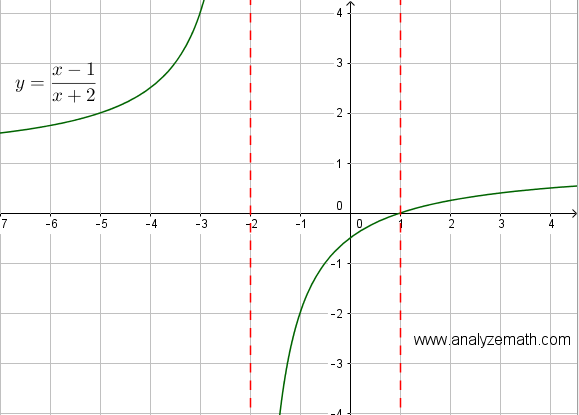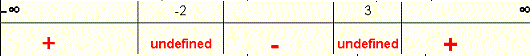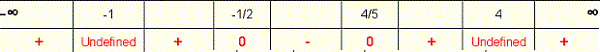# Solving Rational Inequalities Questions with Solutions

Questions with detailed solutions on solving rational inequalities are presented along with detailed solutions. Tables of signs and graphical solutions of some inequalities are also included.

## Review Rational Inequalities

The sign of a rational expression P/Q , where P and Q are polynomials, depends on the signs of P and Q. In turn the signs of P and Q depend on the zeros of P and Q respectively if there are any. Hence the sign of P/Q depends on the zeros of both P and Q and changes (if it does!) only at these zeros. So to solve an inequality of the form P/Q > 0 (or P/Q < 0), we first find the zeros of P and Q then make a table of sign of P/Q.

## solving rational inequalities: Questions with Solutions

Question 1
Solve the rational inequality given byand produce a table of signs and graph the right hand side of the inequality to explain graphically the solution set found analytically.
Solution to Question 1
We first find the zeros of the numerator and the denominator
The numerator is always negative and therefore does not change sign.
Zero of the denominator: solve -x + 4 = 0 to obtain x = 4.
The zero x = 4 divide the real number line into two intervals
(- , 4) , and (4 , + )
We now select and test values that are within each interval and test the rational expressionto find its sign.
a) interval (- , 4)
test value x = 0
We now evaluateat x = 0 to find its sign.(negative).
b) interval (4 , )
test value x = 5
We now evaluateat x = 5 to find its sign.(positive).
We now put all the above results in a table

 x -∞ 4 -∞ - 3 / (- x + 4) - undefined +

Conclusion The solution set of the given rational inequality is given by the interval
(4 , + )

Graphical solution to the given inequality.
Below is shown the graph fo the function y = (-3)/(- x + 4). It is easy to check graphically that y = (-3)/(- x + 4) is positive over the interval
(4 , ).Question 2
Solve the rational inequality given byGraph the right hand side of the inequality to explain graphically the solution set found analytically.
Solution to Question 2
We first find the zeros of the numerator and the denominator
Zero of the numerator: solve x - 1 = 0 to obtain x = 1.
Zero of the denominator: solve x + 2 = 0 to obtain x = -2.
The two zeros x = 1 and x = -2 divide the real number line into three intervals (Note that the zeros are ordered from smallest to the largest).
(- , -2) , (-2 , 1) and (1 , + )
We now select and test values that are within each interval and test the rational expressionto find its sign.
a) interval (- , -2)
test value x = -3
We now evaluateat x = -3 to find its sign.b) interval (-2 , 1)
test value x = 0
We now evaluateat x = 0 to find its sign.c) interval (1 , + )
test value x = 2
We now evaluateat x = 2 to find its sign.Let us now put all the above results in a table
In the interval (- , -2) ,is positive
In the interval (- 2 , 1) ,is negative
In the interval (1 , + ) ,is positive

 -∞ -2 1 ∞ + undefined - 0 +

Conclusion The solution set of the given rational inequality is given by the interval
(- , -2) U (1 , + )

Graphical solution to the given inequality.
Below is shown the graph of the function y = (x-1)/(x+2). It is easy to check graphically that y = (x-1)/(x+2) is positive over the interval
(- , -2) U (1 , + ).Question 3
Produce a table of signs of the inequality given byand determine the solution set.

Solution to Question 3
NOTE: Do not multiply both sides by the LCD (x - 3)(x + 4) as you would do if this was an equation. The sign of (x - 3)(x + 4) changes with x and we do not know if the order of the inequality is to be changed or not.
GivenWrite the inequality with the right side equal to zero by subtracting
3 / (x + 4) from both sides.Rewrite the inequality so that the two terms making the left side have common denominator.Multiply factors, add the two rational expressions on the left side of the inequality and group like terms in the numerator to obtainThe zero of the numerator: - x + 17 = 0 is x = 17
The zeros of the denominator: (x - 3)(x + 4) = 0 are x = 3 and x = -4
The three zeros divide the real number line into four intervals (Note that the zeros are ordered from the smallest to the largest).
(- , -4) , (-4 , 3) , ( 3 , 17) , (17 , +)

a) (- , -4) test value: x = -5
We now evaluate ( -x + 17) / [ (x - 3)(x + 4) ] at x = -5 to find its sign.
( -x + 17) / [ (x - 3)(x + 4) ]
= ( -(-5) + 17) / [ (-5 - 3)(-5 + 4) ]
= 22/8 (positive)
b) (- 4 , 3) test value: x = 0
We now evaluate ( -x + 17) / [ (x - 3)(x + 4) ] at x = 0 to find its sign.
( -x + 17) / [ (x - 3)(x + 4) ]
= ( 0 + 17) / [ (0 - 3)(0 + 4) ]
= -17 / 12 (negative)
c) (3 , 17) test value: x = 4
We now evaluate ( -x + 17) / [ (x - 3)(x + 4) ] at x = 4 to find its sign.
( -x + 17) / [ (x - 3)(x + 4) ]
= (- 4 + 17) / [ (4 - 3)(4 + 4) ]
= 13/8 (positive)
d) (17 , +) test value: x = 18
We now evaluate ( -x + 17) / [ (x - 3)(x + 4) ] at x = 18 to find its sign.
( -x + 17) / [ (18 - 3)(x + 4) ]
= ( -18 + 17) / [ (18 - 3)(18 + 4) ]
= -1/330 (negative)
Let us now put all the above results in a table

 -∞ -4 3 17 -∞ + undefined - undefined + 0 -

Conclusion The solution set of the given rational inequality is given by the interval
(- 4 , 3) U (17 , + )

Question 4
Solve the rational inequality given by

\dfrac{2x + 1}{x+2} \ge \dfrac{x-4}{x - 3}
and include the table of signs of the inequality and the graphical solution.
Solution to Question 4
Given Subtract (x - 4) / (x - 3) from both sides of the given inequality so that its right side equal to zero.
\dfrac{2x + 1}{x+2} - \dfrac{x-4}{x - 3} \ge 0

Rewrite the two rational expressions on the left side with common denominator.Add the two rational expressions and simplify the numerator to obtain
\dfrac{x^2- 3x + 5}{(x+2)(x-3)} \ge 0

Zeros of numerator and denominator
The numerator is a quadratic expression of the form ax2 + bx + c whose discriminant , b2 - 4(a)(c) = (-3)2 - 4(1)(5) = -11 , is negative and therefore has no zeros.
The zeros of the denominator: (x + 2)(x - 3) = 0 are x = -2 and x = 3.
The two zeros divide the real number line into 3 intervals as follows:
(- , -2) , (-2 , 3) and (3, +)

Select test values that are within each of the 3 intervals above and test the rational expression
\dfrac{x^2- 3x + 5}{(x+2)(x-3)}
to find its sign within each interval.
a) interval (- , -2)
test value x = - 3
We now evaluate
\dfrac{x^2- 3x + 5}{(x+2)(x-3)}
at x = -3 to find its sign.

\dfrac{(-3)^2- 3(-3) + 5}{((-3)+2)((-3)- 3)} = \dfrac{23}{6}
which is positive.
b) interval (-2 , 3)
test value x = 0
We now evaluate
\dfrac{x^2- 3x + 5}{(x+2)(x-3)}
at x = 0 to find its sign.

\dfrac{(0)^2- 3(0) + 5}{((0)+2)((0)- 3)} = -\dfrac{5}{6}
which is negative.
c) interval (3 , )
test value x = 4
We now evaluate
\dfrac{x^2- 3x + 5}{(x+2)(x-3)}
at x = 4 to find its sign.

\dfrac{(4)^2- 3(4) + 5}{((4)+2)((4)- 3)} = \dfrac{9}{6}
which is positive.
We now put all the above in a table of sign.Conclusion The solution set of the given rational inequality is given by the interval
(- , -2) U (3 , )

Graphical solution to the inequality.
In the first step above, we obtained the inequality
\dfrac{2x + 1}{x+2} - \dfrac{x-4}{x - 3} \ge 0
which is equivalent to the given inequality. Below is shown the graph to the function
y = \dfrac{2x + 1}{x+2} - \dfrac{x-4}{x - 3}
which represents the left side of the inequality. It is easy to check graphically that y is positive over the interval
(- , -2) U (3 , )
.Question 5
Solve the rational inequality given by

\dfrac{x}{x^2 - 2x - 3} \le \dfrac{1}{x + 3}
including the table of signs of the inequality and the graphical solution.
Solution to Question 5

Subtract 1 / (x + 3) from both sides of the inequality so that its right side equal to zero.

\dfrac{x}{x^2 - 2x - 3} - \dfrac{1}{x + 3} \le 0

Factor the quadratic expression x2 - 2 x - 3 in the denominator.
\dfrac{x}{(x-3)(x+1)} - \dfrac{1}{x + 3} \le 0

Rewrite the rational the two expressions on the left side with the common denominator (x - 3)(x + 1)(x + 3) .Add and simplify the two rational expressions on the left side of the inequality and simplify the numerator to obtain
\dfrac{5x + 3}{(x-3)(x+1)(x+3)} \le 0

Zeros of numerator and denominator
The zero of the numerator: 5x + 3 = 0, x = -3/5.
The zeros of the denominator: (x - 3)(x + 1)(x + 3) = 0 are x = 3, x = -1 and x = -3.
The four zeros divide the real number line into 5 intervals as follows:
(- , -3) , (-3 , -1) , (-1 , -3/5) , (-3/5 , 3) , (3 , +)

Select and test values that are within each interval and test the rational expression
\dfrac{x}{x^2 - 2x - 3} - \dfrac{1}{x + 3}
and find its sign.
a) interval (- , -3)
test value x = -4
We now evaluate
\dfrac{x}{x^2 - 2x - 3} - \dfrac{1}{x + 3}
at x = -4 to find its sign.

\dfrac{(-4)}{(-4)^2 - 2(-4) - 3} - \dfrac{1}{(-4) + 3} = \dfrac{17}{21}
which is positive
b) interval (-3 , -1)
test value x = -2
We now evaluate
\dfrac{x}{x^2 - 2x - 3} - \dfrac{1}{x + 3}
at x = -2
\dfrac{(-2)}{(-2)^2 - 2(-2) - 3} - \dfrac{1}{(-2) + 3} = - \dfrac{7}{5}
which is negative
c) interval (-1 , -3/5)
test value x = -0.8
We now evaluate
\dfrac{x}{x^2 - 2x - 3} - \dfrac{1}{x + 3}
at x = -0.8 to find its sign.

\dfrac{(-0.8)}{(-0.8)^2 - 2(-0.8) - 3} - \dfrac{1}{(-0.8) + 3} \approx 0.6
which is positive
d) interval (-3/5 , 3)
test value x = 0
We now evaluate
\dfrac{x}{x^2 - 2x - 3} - \dfrac{1}{x + 3}
at x = 0 to find its sign.

\dfrac{0}{0^2 - 2(0) - 3} - \dfrac{1}{(0)+ 3} = - \dfrac{1}{3}
which is negative
d) interval (3 , + )
test value x = 4
We now evaluate
\dfrac{x}{x^2 - 2x - 3} - \dfrac{1}{x + 3}
at x = 4 to find its sign.

\dfrac{(4)}{(4)^2 - 2(4) - 3} - \dfrac{1}{(4) + 3} = \dfrac{23}{35}
which is positive.
Let us now put all the above results in a table of signConclusion The solution set of the given rational inequality is given by the interval
(-3 , -1) U [ -3/5 , 3 )

Graphical solution to the inequality.
We obtained above an equivalent (to the given) inequality to solve , which is
\dfrac{x}{x^2 - 2x - 3} - \dfrac{1}{x + 3} \le 0

Below is shown the graph to the function
y = \dfrac{x}{x^2 - 2x - 3} - \dfrac{1}{x + 3}
which represents the left side of the inequality. It is easy to check graphically that y is negative or zero over the interval
(-3 , -1) U [ -3/5 , 3 )
.Question 6
Solve the rational inequality given by

\dfrac{3x^2}{|x^2-3x-4|} \ge \dfrac{1}{3}
including the table of signs of the inequality and the graphical solution.
Solution to Question 6
Write the inequality with the right side equal to zero by subtracting
1 / 3 from both sides.
\dfrac{3x^2}{|x^2-3x-4|} - \dfrac{1}{3} \ge 0

Rewrite the left side of the inequality so that all terms have common denominator.Group
\dfrac{9x^2- |x^2-3x-4|} {3|x^2-3x-4|} \ge 0

The zeros of the numerator are found by solving two equations: (the expression within the absolute value symbol may be positive or negative)
1) 9x2 - (x2 - 3x - 4) = 0
8x2+ 3x + 4 = 0 , discriminant = 32 - 4(8)(4) < 0 , this equation has no real solutions
2) 9x2 - (-1)(x2 - 3x - 4) = 0
10x2 - 3x - 4 = 0 , two solutions: x = -1/2 and x = 4/5.

The zeros of the denominator: x2 - 3x - 4 = 0 are x = - 1 and x = 4

The four zeros divide the real number line into five intervals (Note that the zeros are ordered from the smallest to the largest).

(- , -1) , (-1 , -1/2) , ( -1/2 , 4/5) , ( 4/5 , 4) , (4 , +)

Select test values that are within each interval and use them to find the sign of the expression
\dfrac{3x^2}{|x^2-3x-4|} - \dfrac{1}{3}
which the right hand side of the inequality obtained above in step 1.
a) interval (- , -1)
test value x = - 2
We now evaluate
\dfrac{3x^2}{|x^2-3x-4|} - \dfrac{1}{3}
at x = - 2 to find its sign.

\dfrac{3( - 2)^2}{|( - 2)^2-3( - 2)-4|} - \dfrac{1}{3} = \dfrac{5}{3}
which is positive.
b) interval (-1 , -1/2)
test value x = - 0.75
We now evaluate
\dfrac{3x^2}{|x^2-3x-4|} - \dfrac{1}{3}
at x = - 0.75 to find its sign.

\dfrac{3(- 0.75)^2}{|(- 0.75)^2-3(- 0.75)-4|} - \dfrac{1}{3} \approx 1.09
which is positive.
c) interval (-1/2 , 4/5)
test value x = 0
We now evaluate
\dfrac{3x^2}{|x^2-3x-4|} - \dfrac{1}{3}
at x = 0 to find its sign.

\dfrac{3(0)^2}{|(0)^2-3(0)-4|} - \dfrac{1}{3} = - \dfrac{1}{3}
which is negative
d) interval (4/5 , 4)
test value x = 1
We now evaluate
\dfrac{3x^2}{|x^2-3x-4|} - \dfrac{1}{3}
at x = 1 to find its sign.

\dfrac{3(1)^2}{|(1)^2-3(1)-4|} - \dfrac{1}{3} = \dfrac{1}{6}
which is positive.
d) interval (4 , + )
test value x = 5
We now evaluate
\dfrac{3x^2}{|x^2-3x-4|} - \dfrac{1}{3}
at x = 5 to find its sign.

\dfrac{3(5)^2}{|(5)^2-3(5)-4|} - \dfrac{1}{3} = \dfrac{73}{6}
which is positive.
Let us now put all the above results in a table of sign.• Conclusion The solution set of the given rational inequality is given by the interval
(- , -1) U (-1 , -1/2] U [ 4/5 , 4) U (4 , +)

Graphical solution to the inequality.
Shown below is an equivalent (to the given) inequality to solve which was obtained in step 1.
\dfrac{3x^2}{|x^2-3x-4|} - \dfrac{1}{3} \ge 0

Below is shown the graph to the function
y = \dfrac{3x^2}{|x^2-3x-4|} - \dfrac{1}{3}
which represents the left side of the inequality. It is easy to check graphically that y is positive or zero over the interval
(- , -1) U (-1 , -1/2] U [ 4/5 , 4) U (4 , +)
.